# Vector function

A function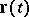of an argumentwhose values belong to a vector space.

A vector function with values in a finite-dimensional (-dimensional) vector spaceis completely determined by its components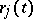,, with respect to some basis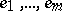of: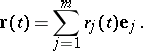(1)

A vector function is said to be continuous, differentiable, etc. (at a point or in a domain) if all functionsare continuous, differentiable, etc. The following formulas are valid for a functionof one variable: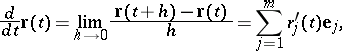(2)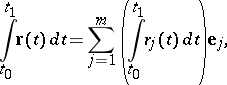(3)(Taylor's formula).

The set of vectors(starting at zero in) is called the hodograph of the vector function. The first derivative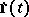of a vector function of one real variable is a vector intangent to the hodograph at the point. Ifdescribes the motion of a point mass, wheredenotes the time, thenis the instantaneous velocity vector of the point at the time. The second derivativeis the acceleration vector of the point.

Partial derivatives and multiple integrals of vector functions of several variables are defined in analogy with formulas (2) and (3). See Vector analysis; Gradient; Divergence; Curl, for the concepts of vector analysis for vector functions.

In an infinite-dimensional normed vector space with a basis, the representation of a vector function in the form (1) is an infinite series, and a coordinate-wise definition of the operations of mathematical analysis involves difficulties connected with the concepts of convergence of series, the possibility of term-by-term differentiation and integration, etc.